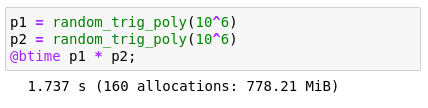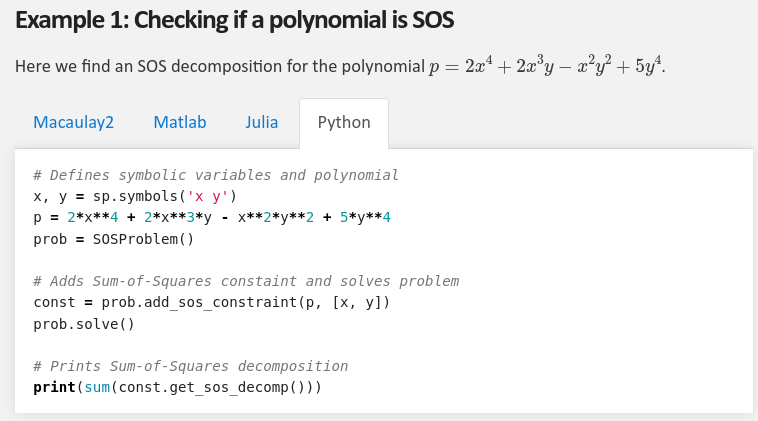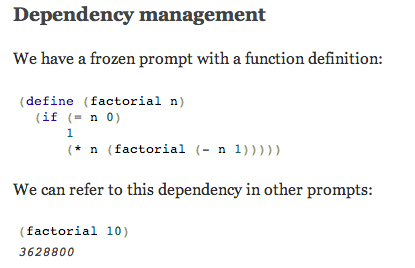Chenyang Yuan
About Research Software Sum of Squares Misc
• ### Software

Some software I created during my undergrad and PhD.

•### TrigPolys.jl

A trignometric polynomial is defined by

$p(x) = a_0 + \sum_{k=1}^n a_k \cos(kx) + a_{-k} \sin(kx)$

The polynomial $$p(x)$$ can be represented either by $$2n+1$$ coefficients $$a_k$$ or by evaluations at $$2n+1$$ distinct points in the interval $$[0,2\pi)$$. This package provides the functions evaluate and interpolate using the Fast Fourier Transform (FFT) to convert efficiently between these two representations. [github] [docs]

•### SumOfSquares.py

Sum of squares modelling language built on top of picos. In addition to modelling convex relaxations of polynomial optimization problems, this package also provides easy access to pseudoexpectation operators for both formulating problems and extracting solutions via rounding algorithms. [github] [docs]

•### coding.js

This is an set of Javascript-based interpreters which use web-workers. It differs from other Javascript interpreters as the main interpreting work is done in a seperate worker thread, and thus would not slow down the main UI thread when performing a lengthy computation. There is a front-end providing the input and output prompts, which links to many separate interpreter backends. Currently the main backend is a Scheme interpreter, which implements a significant subset of Scheme. There is also a logic interpreter based on a subset of Prolog taught in CS61A at Berkeley, and a Javascript interpreter which just calls eval. [github] [demo]